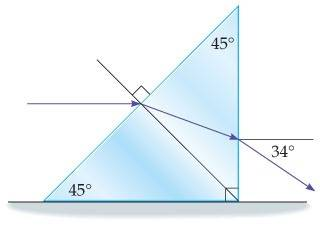## Homework Statement

A horizontal beam of light enters a 45-90-45 prism at the center of it's long side, as shown below. The emerging ray moves in a direction that is 34˚ below the horizontal. What is the index of refraction for the prism?## Homework Equations

n$_{1}$sinø$_{1}$ = n$_{2}$sinø$_{2}$

## The Attempt at a Solution

n1 x sin(i) = n2 x sin(r1): (1st refrection)

and n2 x sin(r2) = n1 x sin(34˚): (2nd refraction)

^^Here n1 is the refractive index of air, n2 is the refractive index of prism, r1 and r2 are the angles of reflection at the two surfaces, and i is the incidence angle.

r1 + r2 = 45˚ or r2 = 45˚ - r1.
Substituting the above value of r2 in the equation(2),we get
n2 x sin(45 - r1) = 1 x sin(34˚)

...and then I'm stuck!

To simplify what I've figured out:
first refraction: sin45 = n sin a

second refraction: n sinb = sin34

and using geometry: a + b = 45

BUT HOW DO I FIND a and b?!?!?

tiny-tim
Homework Helper
Hi ObviousManiac!first refraction: sin45 = n sin a

second refraction: n sinb = sin34

and using geometry: a + b = 45

BUT HOW DO I FIND a and b?!?!?

sin(45° - a) = … ?Hi ObviousManiac!sin(45° - a) = … ?okay so sin(45˚ - a) = sin(45)cos(a) - cos(45)sin(a)

but I'm not sure where to take it from there...
I tried setting up something like:

sin(45)/sin(34) = sin(a)/sin(b)
sin(45)/sin(34) = sin(a)/sin(45-a)
sin(45)/sin(34) = sin(a)/[sin(45)cos(a) - cos(45)sin(a)]

but I don't even know if any of that ^^ is right, I could be going in a totally wrong direction.

tiny-tim
Homework Helper
Hi ObviousManiac!first refraction: sin45 = n sin a
okay so sin(45˚ - a) = sin(45)cos(a) - cos(45)sin(a)

Substitute from the first equation into the second.Hi ObviousManiac!Substitute from the first equation into the second.Sin(45-a) = nsin(a)cos(a) - cos(45)sin(a)

...doesn't this just introduce a new variable? (n?)